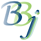# BBjDrawPanel::drawPolygon

## Description

In BBj 16.0 and higher, this method draws a polygon on the BBjDrawPanel using the current pen settings.

## Syntax

 Return Value Method void drawPolygon(java.util.Listlist)

## Parameters

 Variable Description list A list of (x,y) values representing points.

None.

## Remarks

Points are specified as a list (e.g. BBjVector) of numbers `(x1,y1,x2,y2,x3,y3,...)`.

## Example

 rem ' BBjDrawPanel::drawPolygon sysgui = unt open (sysgui)"X0" sysgui! = bbjapi().getSysGui() title\$ = "BBjDrawPanel::drawPolygon" window! = sysgui!.addWindow(25,25,1225,225,title\$,\$00090003\$) window!.setCallback(window!.ON_CLOSE,"eoj") window!.setTrack(0) window!.setFont(sysgui!.makeFont("Arial",8,0)) drawpanel! = window!.getDrawPanel() drawpanel!.setPenColor(BBjColor.BLUE) drawpanel!.setTextColor(BBjColor.BLUE) drawpanel!.setFillColor(BBjColor.GREEN) for i = 0 to 11   x = i * 100 + 25   w = (i+1) * 0.25   drawpanel!.setFillType(i)   drawpanel!.setPattern(i)   drawpanel!.setPenWidth(w)   drawpanel!.drawText(x,25,"setPenWidth("+str(w)+")")   drawpanel!.drawText(x,50,"setPattern("+str(i)+")")   drawpanel!.drawText(x,75,"setFillType("+str(i)+")")   points = i + 3   list! = bbjapi().makeVector()   for point = 0 to points-1     angle = point * 2 * Math.PI / points     px = int(40 * cos(angle) + 25 + x)     py = int(40 * sin(angle) + 150)     list!.add(px)     list!.add(py)   next point   drawpanel!.drawPolygon(list!) next i process_events eoj: release